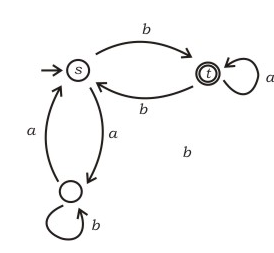Open in App
Not now

# Aptitude | GATE IT 2006 | Question 3

• Last Updated : 28 Jun, 2021

In the automaton below, s is the start state and t is the only final state.Consider the strings u = abbaba, v = bab, and w = aabb. Which of the following statements is true?

(A) The automaton accepts u and v but not w
(B) The automaton accepts each of u, v, and w
(C) The automaton rejects each of u, v, and w
(D) The automaton accepts u but rejects v and w

Explanation:

For the acceptance and rejection of any string we can simply check for the movement on each input alphabet between states. A string is accepted if we stop at any final state of the DFA.
For string u=abbaba the string ends at t (final state) hence it is accepted by the DFA.
For string v=bab the string ends at s (non-final state) and hence rejected by the DFA.
For string w=aabb the string ends at s (non-final state) and hence rejected by the DFA.

This solution is contributed by Yashika Arora.

Quiz of this Question

My Personal Notes arrow_drop_up
Related Articles